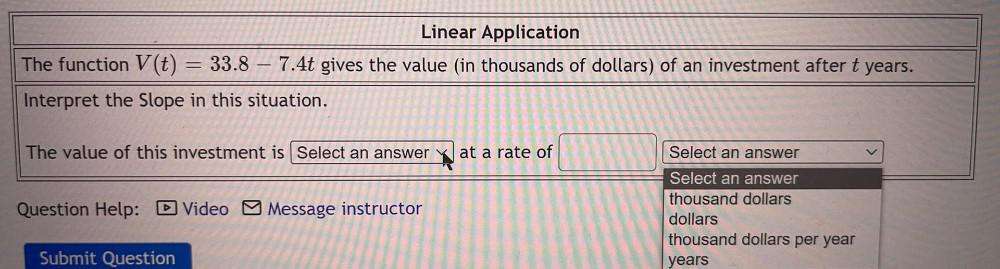Question:

# The function V(t) =33.8 – 7.4t gives the value (in thousands of dollars) of an investment after t years. Interpret the Slope in this situation. The value of this investment is_______ at a rate of_____The function V(t) =33.8 – 7.4t gives the value (in thousands of dollars) of an investment after t years. Interpret the Slope in this situation. The value of this investment is_______ at a rate of_______